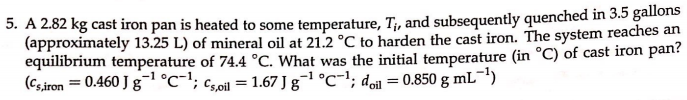# 5. A 2.82 kg cast iron pan is heated to some temperature, Ti, and subsequently quenched in 3.5 gallons (approximately 13.25 L) of mineral oil at 21.2 degrees C to harden the cast iron. The system reaches an equilibrium temperature of 74.4 degrees C. What was the initial temperature (in degrees C) of cast iron pan? (Cs,iron = 0.460 J g^-1 degrees C^-1; Cs,oil = 1.67 J g^-1 degrees C^-1, doil = 0.850 g mL^-1)